SELF 4 S.B. Karavashkin and O.N. Karavashkina6. Citation: ”The point was that Einstein without grounds has applied the computations, which are true for an IRF to a NRF. Try to do so even in frames of non-relativistic mechanics and see what will happen”. “If you apply SR to NRF directly with keeping all postulates, even in Fitzgerald’s initial formulation you will not yield the Lorentz transformation - this means, you will not yield the basis to introduce the light speed constancy”. Even in classical Newtonian mechanics, we can solve the problems in NRF, introducing the related changes to the motion equations - it often is even easier than in IRF. In absence of gravitation, SR deals with IRFs, but one of its principles is also, it is locally applicable to NRFs. This all occurs just as in classical mechanics, with the change of motion equations, but again, locally, i.e. to each point of NRF we put in correspondence its own IRF. We only may not apply SR to the whole NRF. In this case SR would have a weak sense. And GR appears to be an addition to SR of the equivalence principle by the method of which I already said, which was also in SR. So Einstein did nothing discrepant with SR, everything is in limits of SR and equivalence principle. First of all we would like to mark: in our post, as well as in previous posts on this subject, we said not of intrinsic RF which Einstein did not apply when deriving the regularity of light speed against the gravity potential. Both in the paper and in our replies, it was pointed that he illegally and incorrectly made the transition from IRF to NRF. To finish this subject, let us consider this issue in details. For it, let us sequentially follow Einstein’s derivation after his paper “On the gravity force affection on light propagation” . The initial Einstein’s statement of problem was the following: “Let two physical systems of bodies S1 and S2 equipped by measuring devices be located on the axis Z of the system K at the distance h from each other (the sizes of systems S1 and S2 are thought to be infinitesimal in comparison with h), so that the gravity potential at the place where S2 is located is byh more than the gravity potential at the place of S1 location (Fig. 1)” [7, p. 167].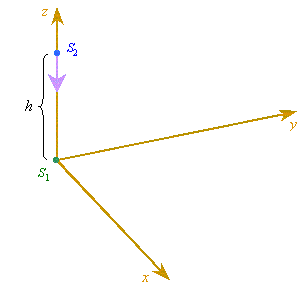Fig. 1
 It follows from this statement, we do not discuss any locality which usually is determined by the constancy of potential in the studied region. Here, on the contrary, it is premised the change of gravity potential at the interval between the source and receiver of light. So the relativistic tricks of which you are saying and which we also know, with whose help SR has been squeezed into GR, are inapplicable to this problem.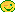At the same time we would mark a considerable incompleteness of this statement of problem; as we will see below, this will tell on further Einstein’s reasoning. From the text we do not see the location of this system in the gravity field, whether it is inertial, its orientation in relation to the field source. Secondly, on one hand, at the points S1 and S2 the gravity potentials are different, which premises the non-uniformly accelerated motion of material bodies in the selected field of space, but from the other hand, further we will see just the uniformly accelerated RF. And thirdly, Einstein wrote the difference of potentials as the producth , whereis not the gravity constant that is usually denoted by this symbol. Using the standard formulation of Newton’s law, we can easily determine that in this case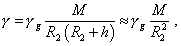(24)
 whereg is the gravity constant, M is the mass of gravitating body, R2 is the distance from the gravitating body to S2 . Thus, it follows from (24) that, according to Einstein’s record, the difference of potentials has to be very small; this means that the derived formula is true only in the region of weak fields or small distances between the source and receiver, asis the function of distance to the gravitating body. In violation of this condition we have to consider a quite nonlinear problem with other statement. Let us go on through the text of derivation of this regularity. At the next stage Einstein, as expected, sends the ray of light from S2 to S1 and here first difficulties of the logic begin. Einstein writes: “A priori we can say nothing of the process of energy transfer with radiation, because we do not know, how the field of gravity force affects the radiation energy and the measuring instruments in S1 and S2 ” [7, p. 168]. Basically, according to the common scientific logic, at this point we should stop the further derivation of the light speed regularity with respect to the potential, until we clear the regularity of above affection. However, Einstein ignores this basic principle of study and goes on: “But in accordance with the admitted equivalence of reference frames K and K' , we can, instead the frame K located in homogeneous (? - Authors) gravity field, put a gravity-free reference frame K' moving with the uniform acceleration towards the positive z, with whose axis the physical systems S1 and S2 are strongly constrained” [7, p. 168]. As we can see, there was made a highly wrong substitution when developing of the statement of problem. If the gravity field was homogeneous, the difference of potentials between S1 and S2 will be absent; then the motion of equivalent RF applied to a very limited case of radial location of S1 and S2 can be thought as uniformly accelerated. Though, only if the gravity field affects the light to the same extent as material bodies. If the gravity field did not affect the light ray at all, the equivalence introduced by Einstein becomes groundless. The light ray will propagate between S1 and S2 in the same way both in gravity field and out of it. The same in partial interaction of the field with light. The substitution will be true only if the gravity field affects the light as a material body, and S1 and S2 are in the field of same potential. At the same time, Einstein admitted impossible to substantiate such statement of problem a priori. We can add, Eddington’s study of stellar light deflection  may not be taken as the grounds for Einstein’s premises.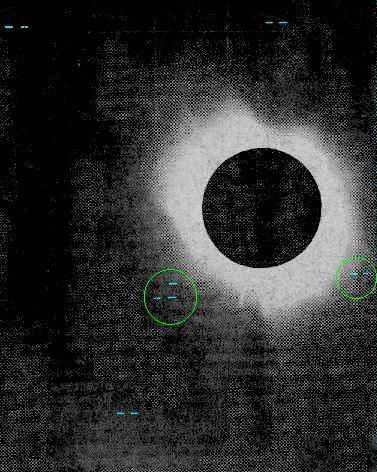Fig. 2. The positive image from the half-tone reproduction of one of negatives obtained with 4-inch objective in Sobrale. The stars location is marked [8, p. 569]
 In the shown image the ray tracks are strongly horizontal. At the same time, noting that the Earth displaces in time, the ray location with respect to Sun also changes. With it, the photographic plates would have to register both radial and horizontal shift of the ray. Thereupon, in presence of interaction of light with the gravity field, the ray tracks have to be inclined to the radius. This especially concerns the stellar groups whose rays pass close to the Sun crown. We have to add, similar studies during the Sun eclipse of 1922 showed basically different result shown in Fig. 3 [10, p. 566].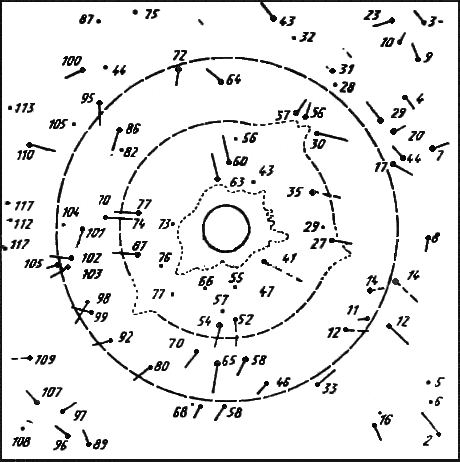Fig. 3. The shift of images of stars on the photographic plates in observations during the Sun eclipse of 1922
 In the shown Figure, the stellar tracks are even more chaotic. Some of tracks are directed to the Sun, some other - from the Sun. In the right bottom corner, the 16th star is shifted against that 2nd, in the left we can see the opposite shift in the stars 74 and 77; in the right - in the stars 44 and 16, 29 and 20; in the right top corner - stars 10 and 23, in the top centre - stars 72 and 66. The shift of the star 101 is normal to that of the near stars 102, 103 and 105 in the right. Such chaos is in all regions of the image, and only conventionally we can call the stellar shift radial. But even such shift is discrepant with the phenomenology of interaction of light with the gravity field in case when we neglect the refraction. The expected pattern has to be shown as in Fig. 4.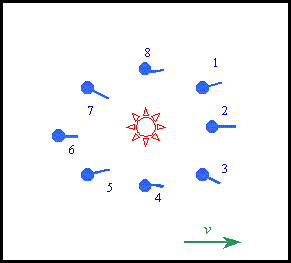Fig. 4. The pattern of stellar shift in the gravity field of Sun in case of gravitation affection on the light
 We see in Fig. 4 that stars 2 and 6 being on the axis parallel to the Earth’s shift and passing through the centre of Sun have to have a horizontal shift. The tracks of stars 1 and 3 have to be directed outwards, and those of stars 5 and 7 – inwards, because due to the Earth’s shift we have to see the rays passing, correspondingly, through the weaker and stronger fields. Finally, the tracks of stars 4 and 8 have to be curved, as the part of rays we will register in the growing gravity field and another part - in decreasing field. And should these images reflect the shown regularity, we could speak of the interaction of light with the gravity field. Furthermore, we see from Fig. 4 that the gravity interaction with the light can be determined not by the common length of stellar track but only by the track inclination. Thus, the interaction is determined not by the shift value but by the track inclination, which considerably changes the approach to the observation and processing the results. But this regularity, as well as the typical regularity of the stellar track inclination, is seen neither in photos of 1922 nor in Eddington’s photos. On the contrary, the stellar tracks in Fig. 3 are more like refraction of light in the turbulent high-temperature atmosphere of Sun than the light - gravity field interaction. At the same time, it is known that in preparation of calculations for his experiment, Eddington simply neglected the affection of Sun atmosphere, while in considerable temperature gradient and large distance that rays passed near the Sun, these factors had to be carefully calculated and given with the substantiation of experimental method. Instead, we can read in the report: “To obtain the observed effect on the account of refraction, it is necessary, the Sun to be surrounded by the substance with the refraction factor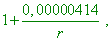(25)
 where r is the distance from the centre in the units of Sun radius. At the height of one radius from the surface, the required refraction factor 1,00000212 corresponds to that of air refraction under the pressure of 1/140, hydrogen - under pressure of 1/60 either helium - under pressure of 1/20 of that atmospheric” [8, p. 566]. From this citation we see that the formula on whose basis they calculated does not include the temperature dependence on Sun atmosphere, the more on considerable temperature gradients; the feature of celestial body at the edge of Sun disk equivalent to the refraction observation when the source is located at the very horizon. This last factor is especially important from the view that “at the very horizon, the light refraction grows with z (zenith distance of celestial body - Authors) so fast that the lower edge of disks of Sun and Moon happens to be slightly raised by several arc minutes more than that upper” [11, p. 443]. With account of these factors, Eddington’s refraction data will considerably grow and will be quite comparable with the effect calculated by Einstein’s formula, which was trice re-calculated, as it was inconsistent with the preliminary results. And this also was reflected in the report of Eddington’s expedition. Thus, we see, it is impossible to rely on the existing observation results of stellar shifts because of the Sun atmosphere’s large masking effects of unthorough metrological provision of the experimental methods.

Contents: / 1 / 2 / 3 / 4 / 5 / 6 / Paper